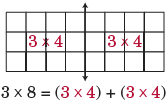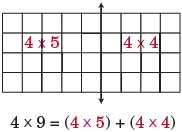# Distributive property

The distributive property can be expressed as:

 a × (b + c) = (a × b) + (a × c)
Examples
 a × (b + c) = (a × b) + (a × c) 3 × (4 + 2) = (3 × 4) + (3 × 2)
In some cases, the distributive property can be used to simplify multiplication facts:
Ex 1
 3 × 8 = 3 × (4 + 4) = (3 × 4) + (3 × 4) = 12 + 12 = = 24Ex 2
 4 × 9 = 4 × (5 + 4) = (4 × 5) + (4 × 4) = 20 + 16 = = 36The distributive property can also be used to help simplify the multiplication of numbers with more than one digit:

Example

It can also be used to help with dividing numbers: